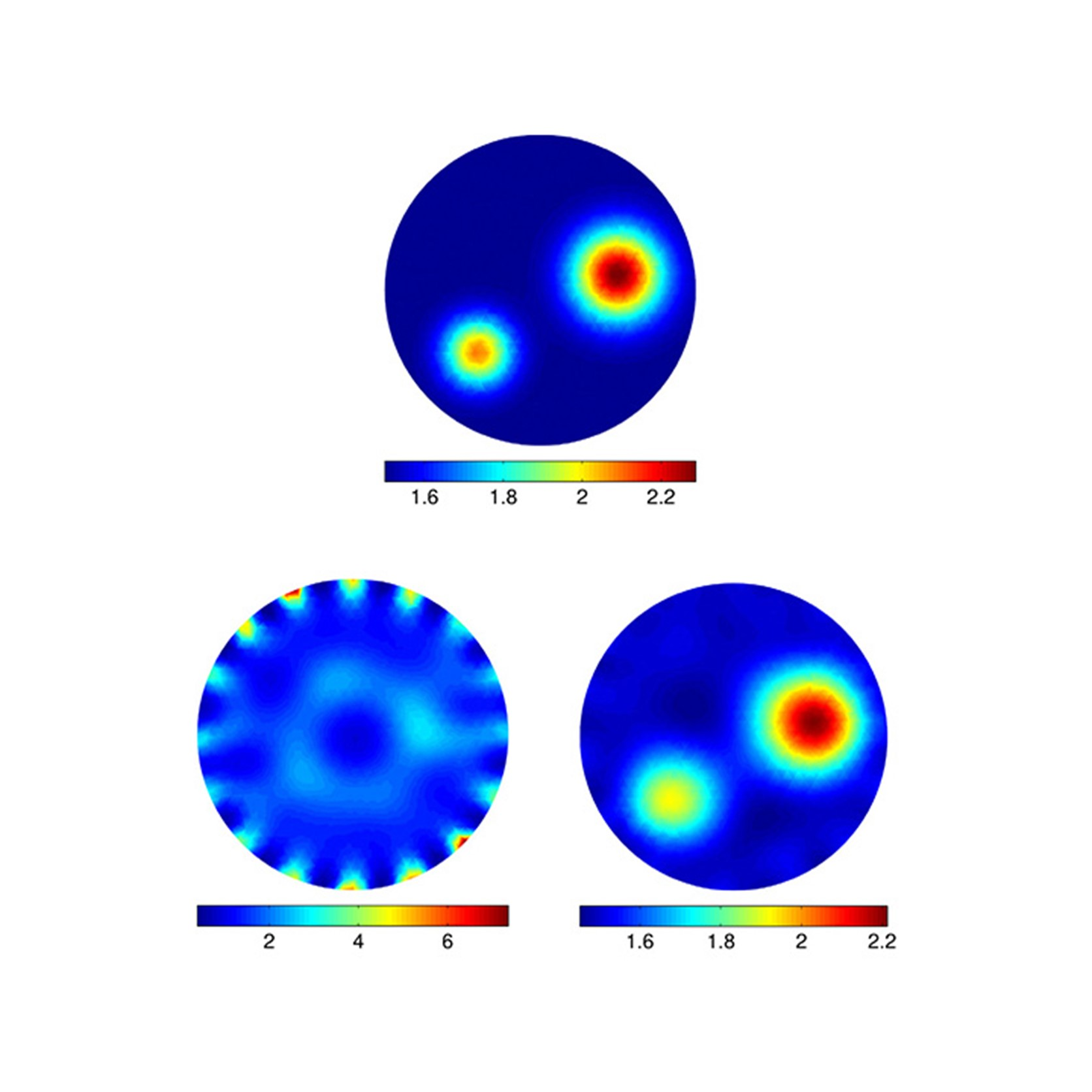# Model Error Reduction in Inverse Problems

Puyuan Mi: How to reduce the error introduced when modelling an inverse problem?

Inverse problems are mathematical problems that arise when one wants to recover "hidden" information from indirect and incomplete measurements/data. The field of inverse problems includes engineering, geoscience, medicine, biology, physics, chemistry, finance, etc. When we solve an inverse problem, we compute the source that gives rise to the observed data, using a mathematical model for the relation between the source and the data. An intrinsic difficulty in inverse problems is the inherent illposedness or instability, which is reflected in the fact that solutions are very sensitive to noise and errors. The influence of measurement noise on the solution, and the corresponding regularization methods for noise suppression, have been extensively studied and is well described – and is still a topic of current research for challenging modalities and noisy types. However, the situation in the case of model errors is rather different.

Model errors are the uncertainty that could be possibly introduced when formulating the model. There are many sources of uncertainty regarded as model errors. Some parameters, like the deposition velocity of radiological materials when estimating properties of a nuclear leak through environmental detections, are difficult to determine and would lead to parameter uncertainty. Sometimes parameters are just variable, like the body position when one is taking a CT scan. Also, as is always believed, no model is perfect, especially for inverse problems. Certain techniques such as discretization or linearization are always applied to guarantee the existence and uniqueness of solutions as well as to make the computation tractable, which would be bound to introduce model errors.

In this project, we will scrutinize the influence of various kinds of model errors and how they influence solutions to inverse problems. This insight will be used to provide a better understanding of the errors in solutions and guide the improvement of the mathematical/computational models and the computed solutions.

A thorough understanding of the influence of model errors must be based on theoretical mathematical studies. We will utilize uncertainty quantification to study the propagation of uncertainty in models and data to the solutions, which will give us further insight to reduce the errors. Based on our theoretical results, we will develop algorithms for efficient and robust handling of the necessary compensations for the model/measurement errors.

The figure is Figure 4 of Daniela Calvetti, Matthew Dunlop, Erkki Somersalo and Andrew Stuart’s paper: Iterative updating of model error for Bayesian.

## PhD project

By: Puyuan Mi

Section: Scientific Computing

Principal supervisor: Yiqiu Dong

Co-supervisor: Bangti Jin

Project title: Model Error Reduction in Inverse Problems

Term: 01/11/2021 → 31/10/2024

## ContactPuyuan Mi
PhD student
DTU Compute

## ContactYiqiu Dong
Associate Professor
DTU Compute
+45 45 25 31 08
https://www.compute.dtu.dk/english/phd/current-phd/phd-sc/puyuan-mi
7 DECEMBER 2023